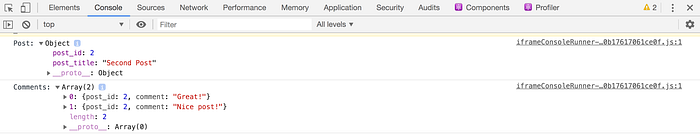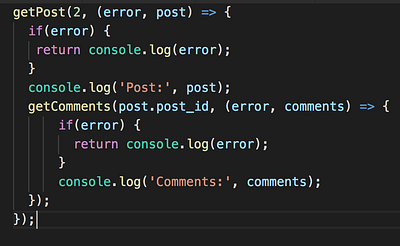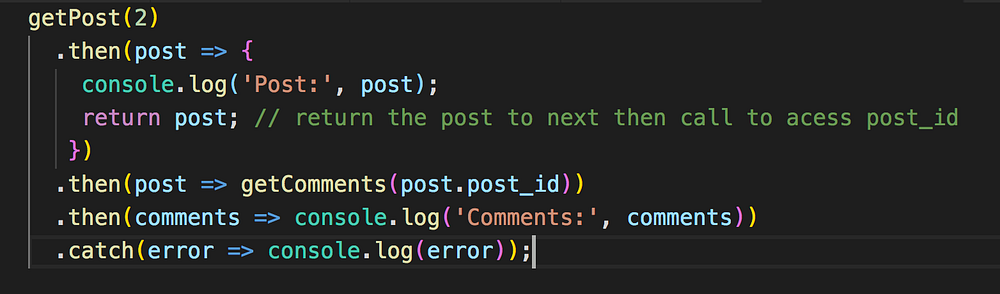# Yogesh Chavan# ES6 Promises: A Better Way of Handling Callbacks

ES6 introduced promises as a native implementation. Before ES6 we were using callbacks to handle asynchronous operations.

In this article, we'll understand what is callback and what problem related to the callback is solved by promises.

Want to learn Redux in detail and build a complete food ordering app? check out my Mastering Redux course. Following is the preview of the app, we'll be building in the course. It's a great project you can add to your portfolio/resume.

Consider, we have a list of posts and their respective comments.

``````const posts = [
{ post_id: 1, post_title: 'First Post' },
{ post_id: 2, post_title: 'Second Post' },
{ post_id: 3, post_title: 'Third Post' },
];

{ post_id: 2, comment: 'Great Post!'},
{ post_id: 2, comment: 'Nice Post!'},
{ post_id: 3, comment: 'Awesome Post!'},
];
``````

We will write a function to get the post by passing post id and if the post is found we will get the comments related to that post

``````const getPost = (id, callback) => {
const post = posts.find( post => post.post_id === id);
if(post) {
callback(null, post);
} else {
callback("No such post", undefined);
}
};

const getComments = (post_id, callback) => {
const result = comments.filter( comment => comment.post_id === post_id);
if(result) {
callback(null, result);
} else {
}
}
``````

Here, we're using array find and filter method to find the post and comment. If you're not familiar with them, check out my this article for a detailed explanation of the most useful array methods.

In the above `getPost` and `getComments` functions, if there is an error we will pass the error message as the first argument to the callback function and if we got the result we will pass the result as the second argument to the callback function.

If you are familiar with Node.js, then you will know that this is a very common practice used in every Node.js callback function.

Now let's use the above functions.

``````getPost(2, (error, post) => {
if(error) {
return console.log(error);
}
console.log('Post:', post);
if(error) {
return console.log(error);
}
});
});
``````

Here, we're calling the `getPost` function by passing 2 for the post id as the first argument and callback function as the second argument.

After execution of the above code, you will see the following output:Here's a Code Pen Demo.

As you can see, we have `getComments` function nested inside `getPost` callback. Imagine if we also want to find the likes of comment then that will also get nested inside `getComments` callback so creating more nesting which will make code difficult to understand.

This nesting of callback is known as callback hell.

Also, you can see that the error handling condition gets repeated which creates a duplicate code which is not good.

So to fix this problem promises were introduced.

To create a new promise we use the Promise constructor like this:

``````const promise = new Promise((resolve, reject) => {

});
``````

The Promise constructor accepts a function as the first parameter.

The `resolve` and `reject` are functions that are automatically passed to the function.

The promise goes through three states.

• Pending
• Fulfilled
• Rejected

When we create a promise, it's in a pending state, and when we call the `resolve` function, it goes in fulfilled state and if we call the `reject` function, it will go in the rejected state.

We perform the asynchronous operation inside the function passed to Promise constructor and when we get the response of asynchronous operation and the response is ok then we call `resolve` function and if there is some error then we call the `reject` function.

``````const resolvedPromise = () => {
return new Promise((resolve, reject) => {
resolve('worked!');
});
};
``````

To access the value of the resolved promise, we need to attach the `.then` handler which will be called when the promise is resolved.

``````resolvedPromise().then((result) => {
console.log(result);  // worked!
});
``````

When the promise gets rejected, the `catch` handler will be executed

``````const rejectedPromise = () => {
return new Promise((resolve, reject) => {
reject('something went wrong!');
});
};

rejectedPromise().catch((error) => {
console.log('Error', error); // Error something went wrong!
});
``````

Note that, we can pass only a single value to resolve and reject function.

Take a look at the below code:

``````const multiply = number => {
if(number > 0) {
return number * number;
} else {
return "Error while multiplying";
}
};

const getPromise = value => {
return new Promise((resolve, reject) => {
const result = multiply(value);
if(typeof result === "number") {
resolve(result);
} else {
reject(result)
}
});
};

getPromise(4)
.then(result => console.log(result)) // 16
.catch(error => console.log(error));

getPromise(-5)
.then(result => console.log(result))
.catch(error => console.log(error)); // Error while multiplying
``````

Here, in the `getPromise` function we are passing some value. If the value is a number then we return the multiplication of number with itself otherwise returns an error.

Here's a Code Pen Demo.

We can also attach multiple `.then` handlers.

``````getPromise(4)
.then(result => {
return getPromise(result);
})
.then(output => console.log('result', output))
.catch(error => console.log('error', error));
``````

The value returned from the first `.then` call will be passed to the second `.then` call and so on.

This way of attaching multiple `.then` calls is known as promise chaining.

Here's a Code Pen Demo.

If any one of the promise in the promise chain gets rejected, the next promise in the chain will not be executed, instead, the execution stops there and the `.catch` handler will be executed.

``````const multiply = number => {
if(number > 0) {
return number * number;
} else {
return "Error while multiplying";
}
};

const getPromise = value => {
return new Promise((resolve, reject) => {
const result = multiply(value);
if(typeof result === "number") {
resolve(result);
} else {
reject(result)
}
});
};

getPromise(4)
.then(result => {
console.log('first');
return getPromise(result);
})
.then(result => {
console.log('second');
return getPromise(-5); // passing negative value will call reject
})
.then(result => { // this will not be executed
console.log('third');
return getPromise(2);
})
.then(output => console.log('last:', output))
.catch(error => console.log('error:', error));
``````

Here's a Code Pen Demo.

As you can see here, only the first and second `.then` block is executed and the third is skipped as the promise gets rejected because of the negative value check in the multiply function.

So now, you have a good understanding of promises and promise chaining, let's see how we can fix the callback hell problem for the first post and comment example using promise chaining.

``````const posts = [
{ post_id: 1, post_title: 'First Post' },
{ post_id: 2, post_title: 'Second Post' },
{ post_id: 3, post_title: 'Third Post' },
];

{ post_id: 2, comment_id: 1, comment: 'Great Post!'},
{ post_id: 2, comment_id: 2, comment: 'Nice Post!'},
{ post_id: 3, comment_id: 3, comment: 'Awesome Post!'},
];

const getPost = (id) => {
return new Promise((resolve, reject) => {
const post = posts.find( post => post.post_id === id);
if(post) {
resolve(post);
} else {
reject("No such post");
}
});
};

const getComments = (post_id) => {
return new Promise((resolve, reject) => {
const result = comments.filter( comment => comment.post_id === post_id);
if(result) {
resolve(result);
} else {
}
});
};

getPost(2)
.then(post => {
console.log('Post:', post);
return post; // return the post to next then call to acess post_id
})
.catch(error => console.log(error));
``````

Here's a Code Pen Demo.

If you compare the code of callback and promise, you can see the difference as shown below.

Callback Code:Promise Code:As you can see, the code using promises is looking easy to understand and clean.

Here, we have changed the callback function call to `resolve` or `reject` depending on response and used promise chaining to avoid the nesting of function calls.

We also avoided duplicate error condition checks using just a single `.catch` handler in promise chaining.

Check out my recently published Mastering Redux course.

In this course, you will learn:

• How to manage the complex state of array and objects
• How to use multiple reducers to manage complex redux state
• How to debug Redux application
• How to use Redux in React using react-redux library to make your app reactive.
• How to use redux-thunk library to handle async API calls and much more

and then finally we'll build a complete food ordering app from scratch with stripe integration for accepting payments and deploy it to the production.

So click the below image to get the course at just \$12 instead of the original price of \$19 only valid till 19th May 2021.

You will also get a free copy of my popular Mastering Modern JavaScript book If you purchase the course till 19th May 2021.Want to stay up to date with regular content regarding JavaScript, React, Node.js? Follow me on LinkedIn.